﻿ n次直纹曲面及其分类 n-Order Ruled Surfaces and Their Classification

Pure Mathematics
Vol. 08  No. 05 ( 2018 ), Article ID: 26796 , 8 pages
10.12677/PM.2018.85070

n-Order Ruled Surfaces and Their Classification

Jixing Wang*, Yue Zhao

School of Mathematics and Statistics, Yunnan University, Kunming Yunnan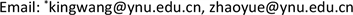Received: Aug. 21st, 2018; accepted: Sep. 6th, 2018; published: Sep. 13th, 2018ABSTRACT

Firstly, this article presents the general expression of n-order ruled surface, and a sufficient and necessary condition for a surface to be a ruled surface. Secondly, it introduces the conceptions of minimum and tight rectilinear generators families, and obtains some interesting results. Lastly, it further studies ruled surfaces and puts them into two classes: compound and inseparable ruled surfaces, and obtains some important properties.

Keywords:Ruled Surface, n-Order Ruled Surface, Compound Ruled Surface, Minimum Rectilinear Generators Family, Tight Rectilinear Generators Family

n次直纹曲面及其分类1. 引言

2. n次直纹曲面

$\underset{s=0}{\overset{n}{\sum }}\underset{\begin{array}{l}\text{}i,j\\ \text{}i+j\le s\end{array}}{\sum }{a}_{\left(i,j,s-i-j\right)}{x}^{i}{y}^{j}{z}^{s-i-j}=0$ (1)

$F\left(x,y,z\right)=\underset{s=0}{\overset{n}{\sum }}\underset{\begin{array}{l}\text{}i,j\\ \text{}i+j\le s\end{array}}{\sum }{a}_{\left(i,j,s-i-j\right)}{x}^{i}{y}^{j}{z}^{s-i-j}$ (2)

${\delta }_{k}\left(s,l,m,r\right)=\left\{\begin{array}{l}1,\text{}s-l-m-r=k\\ 0,\text{}其他\end{array}$

$\left\{\begin{array}{l}x={x}_{0}+Xt\\ y={y}_{0}+Yt\\ z={z}_{0}+Zt\end{array}$

$\begin{array}{l}F\left({x}_{0}+Xt,\text{}{y}_{0}+Yt,\text{}{z}_{0}+Zt\right)\\ =\underset{\text{}s=0}{\overset{n}{\sum }}\underset{\begin{array}{l}\text{}i,j\\ \text{}i+j\le s\end{array}}{\sum }{a}_{\left(i,j,s-i-j\right)}{\left({x}_{0}+Xt\right)}^{i}{\left({y}_{0}+Yt\right)}^{j}{\left({z}_{0}+Zt\right)}^{s-i-j}\\ =\underset{\text{}s=0}{\overset{n}{\sum }}\underset{\begin{array}{l}\text{}i,j\\ \text{}i+j\le s\end{array}}{\sum }{a}_{\left(i,j,s-i-j\right)}\underset{l=0}{\overset{i}{\sum }}{C}_{i}^{l}{x}_{0}^{l}{\left(Xt\right)}^{i-l}\underset{m=0}{\overset{j}{\sum }}{C}_{j}^{m}{y}_{0}^{m}{\left(Yt\right)}^{j-m}\underset{r=0}{\overset{s-i-j}{\sum }}{C}_{s-i-j}^{r}{z}_{0}^{r}{\left(Zt\right)}^{s-i-j-r}\\ =\underset{\text{}s=0}{\overset{n}{\sum }}\underset{\begin{array}{l}\text{}i,j\\ \text{}i+j\le s\end{array}}{\sum }{a}_{\left(i,j,s-i-j\right)}\underset{l=0}{\overset{i}{\sum }}\underset{m=0}{\overset{j}{\sum }}\underset{r=0}{\overset{s-i-j}{\sum }}{C}_{i}^{l}{C}_{j}^{m}{C}_{s-i-j}^{r}{x}_{0}^{l}{y}_{0}^{m}{z}_{0}^{r}{X}^{i-l}{Y}^{j-m}{Z}^{s-i-j-r}{t}^{i-l+j-m+s-i-j-r}\end{array}$ (3)

$\begin{array}{l}=\underset{\text{}s=0}{\overset{n}{\sum }}\underset{\begin{array}{l}\text{}i,j\\ \text{}i+j\le s\end{array}}{\sum }{a}_{\left(i,j,s-i-j\right)}\underset{l=0}{\overset{i}{\sum }}\underset{m=0}{\overset{j}{\sum }}\underset{r=0}{\overset{s-i-j}{\sum }}{C}_{i}^{l}{C}_{j}^{m}{C}_{s-i-j}^{r}{x}_{0}^{l}{y}_{0}^{m}{z}_{0}^{r}{X}^{i-l}{Y}^{j-m}{Z}^{s-i-j-r}{t}^{s-l-m-r}\\ =\underset{k=0}{\overset{n}{\sum }}{G}_{k}\left({x}_{0},{y}_{0},{z}_{0},X,Y,Z\right){t}^{k}\\ =\underset{k=1}{\overset{n}{\sum }}{G}_{k}\left({x}_{0},{y}_{0},{z}_{0},X,Y,Z\right){t}^{k}\end{array}$${G}_{k}\left({x}_{0},{y}_{0},{z}_{0},X,Y,Z\right)=0$

$\left\{\begin{array}{l}x={x}_{0}+Xt\\ y={y}_{0}+Yt\\ z={z}_{0}+Zt\end{array}$

$F\left({x}_{0}+Xt,\text{}{y}_{0}+Yt,\text{}{z}_{0}+Zt\right)=\underset{k=1}{\overset{n}{\sum }}{G}_{k}\left({x}_{0},{y}_{0},{z}_{0},X,Y,Z\right){t}^{k}=0$

(充分性)若对曲面上任意一点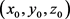都存在一个对应的方向 $\left\{X,Y,Z\right\}$ 使得对任给的 $k=1,2,\cdots ,n$ ，有 ${G}_{k}\left({x}_{0},{y}_{0},{z}_{0},X,Y,Z\right)=0$

$F\left({x}_{0}+Xt,\text{}{y}_{0}+Yt,\text{}{z}_{0}+Zt\right)=\underset{k=1}{\overset{n}{\sum }}{G}_{k}\left({x}_{0},{y}_{0},{z}_{0},X,Y,Z\right){t}^{k}=0$ ，这说明

${M}_{0}=\left({x}_{0},{y}_{0},{z}_{0}\right)$ 是曲面上任意点，且过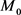的直线方程为

$\left\{\begin{array}{l}x={x}_{0}+Xt\\ y={y}_{0}+Yt\\ z={z}_{0}+Zt\end{array}$

$\begin{array}{l}F\left({x}_{0}+Xt,\text{}{y}_{0}+Yt,\text{}{z}_{0}+Zt\right)\\ =\left[{\left({x}_{0}+Xt\right)}^{2}-{\left({y}_{0}+Yt\right)}^{2}-2\left({z}_{0}+Zt\right)\right]\left[{\left({x}_{0}+Xt\right)}^{2}+{\left({y}_{0}+Yt\right)}^{2}-1\right]\\ =\left[{x}_{0}^{2}+{X}^{2}{t}^{2}+2{x}_{0}Xt-{y}_{0}^{2}-{Y}^{2}{t}^{2}-2{y}_{0}Yt-2{z}_{0}-2Zt\right]\\ \text{\hspace{0.17em}}\text{\hspace{0.17em}}\text{ }×\left[{x}_{0}^{2}+{X}^{2}{t}^{2}+2{x}_{0}Xt+{y}_{0}^{2}+{Y}^{2}{t}^{2}+2{y}_{0}Yt-1\right]\\ =\left[\left({X}^{2}-{Y}^{2}\right){t}^{2}+2\left({x}_{0}X-{y}_{0}Y-Z\right)t+\left({x}_{0}^{2}-{y}_{0}^{2}-2{z}_{0}\right)\right]\\ \text{\hspace{0.17em}}\text{\hspace{0.17em}}\text{ }×\left[\left({X}^{2}+{Y}^{2}\right){t}^{2}+2\left({x}_{0}X+2{y}_{0}Y\right)t+\left({x}_{0}^{2}+{y}_{0}^{2}-1\right)\right]\end{array}$

$\begin{array}{l}=\left({X}^{2}-{Y}^{2}\right)\left({X}^{2}+{Y}^{2}\right){t}^{4}+2\left({x}_{0}X-{y}_{0}Y-Z\right)\left({X}^{2}+{Y}^{2}\right){t}^{3}\\ \text{\hspace{0.17em}}\text{\hspace{0.17em}}+\left({x}_{0}^{2}-{y}_{0}^{2}-2{z}_{0}\right)\left({X}^{2}+{Y}^{2}\right){t}^{2}+2\left({X}^{2}-{Y}^{2}\right)\left({x}_{0}X+2{y}_{0}Y\right){t}^{3}\\ \text{\hspace{0.17em}}\text{\hspace{0.17em}}+4\left({x}_{0}X-{y}_{0}Y-Z\right)\left({x}_{0}X+2{y}_{0}Y\right){t}^{2}+2\left({x}_{0}^{2}-{y}_{0}^{2}-2{z}_{0}\right)\left({x}_{0}X+2{y}_{0}Y\right)t\\ \text{\hspace{0.17em}}\text{\hspace{0.17em}}+\left({X}^{2}-{Y}^{2}\right)\left({x}_{0}^{2}+{y}_{0}^{2}-1\right){t}^{2}+2\left({x}_{0}X-{y}_{0}Y-Z\right)\left({x}_{0}^{2}+{y}_{0}^{2}-1\right)t\\ \text{\hspace{0.17em}}\text{\hspace{0.17em}}+\left({x}_{0}^{2}-{y}_{0}^{2}-2{z}_{0}\right)\left({x}_{0}^{2}+{y}_{0}^{2}-1\right)\end{array}$ (4)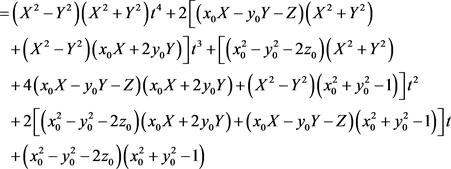${G}_{4}\left({x}_{0},{y}_{0},{z}_{0},X,Y,Z\right)=\left({X}^{2}-{Y}^{2}\right)\left({X}^{2}+{Y}^{2}\right)$

${G}_{3}\left({x}_{0},{y}_{0},{z}_{0},X,Y,Z\right)=2\left[\left({x}_{0}X-{y}_{0}Y-Z\right)\left({X}^{2}+{Y}^{2}\right)+\left({X}^{2}-{Y}^{2}\right)\left({x}_{0}X+2{y}_{0}Y\right)\right]$

${G}_{2}\left({x}_{0},{y}_{0},{z}_{0},X,Y,Z\right)=\left({x}_{0}^{2}-{y}_{0}^{2}-2{z}_{0}\right)\left({X}^{2}+{Y}^{2}\right)+4\left({x}_{0}X-{y}_{0}Y-Z\right)\left({x}_{0}X+2{y}_{0}Y\right)\text{ }+\left({X}^{2}-{Y}^{2}\right)\left({x}_{0}^{2}+{y}_{0}^{2}-1\right)$

${G}_{1}\left({x}_{0},{y}_{0},{z}_{0},X,Y,Z\right)=2\left[\left({x}_{0}^{2}-{y}_{0}^{2}-2{z}_{0}\right)\left({x}_{0}X+2{y}_{0}Y\right)+\left({x}_{0}X-{y}_{0}Y-Z\right)\left({x}_{0}^{2}+{y}_{0}^{2}-1\right)\right]$

${G}_{0}\left({x}_{0},{y}_{0},{z}_{0},X,Y,Z\right)=\left({x}_{0}^{2}-{y}_{0}^{2}-2{z}_{0}\right)\left({x}_{0}^{2}+{y}_{0}^{2}-1\right)=F\left({x}_{0},{y}_{0},{z}_{0}\right)=0$

i) 若 ${x}_{0}^{2}+{y}_{0}^{2}-1=0$

$\left({X}^{2}+{Y}^{2}\right){t}^{2}+2\left({x}_{0}X+{y}_{0}Y\right)t=0$

${G}_{4}\left({x}_{0},{y}_{0},{z}_{0},X,Y,Z\right)={G}_{3}\left({x}_{0},{y}_{0},{z}_{0},X,Y,Z\right)={G}_{2}\left({x}_{0},{y}_{0},{z}_{0},X,Y,Z\right)={G}_{1}\left({x}_{0},{y}_{0},{z}_{0},X,Y,Z\right)=0$ (5)

ii) 若 ${x}_{0}^{2}-{y}_{0}^{2}-2{z}_{0}=0$

$\left\{\begin{array}{l}{X}^{2}-{Y}^{2}=0\\ {x}_{0}X-{y}_{0}Y-Z=0\end{array}$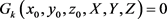3. 直母线族

i) L中的每一条直线都在 $\Sigma$ 上；

ii) 对曲面 $\Sigma$ 上的任意一点，在L中都至少有一条直线经过它。

$\underset{{M}_{{}_{0}}\in \Sigma }{\cup }L\left({M}_{0}\right)$ 就是曲面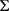的最大直母线族。(下面证明唯一性)设有另一个最大直母线族L，可证明

$\underset{{M}_{{}_{0}}\in \Sigma }{\cup }L\left({M}_{0}\right)=L$ 。事实上，任取 $l\in \underset{{M}_{{}_{0}}\in \Sigma }{\cup }L\left({M}_{0}\right)$ ，由于L是最大直母线族，故 $l\in L$ ，因此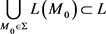${L}_{1}=\left\{\left\{\begin{array}{l}y=a\\ x=0\end{array},\text{}a<0\right\}$${L}_{2}=\left\{\left\{\begin{array}{l}z=a\\ x=0\end{array},\text{}a<1\right\}$${L}_{3}=\left\{\left\{\begin{array}{l}z=1-y+a\\ x=0\end{array},\text{}a\ge 0\right\}$

${L}_{1}\cup {L}_{2}\cup {L}_{3}$ 就是平面 $x=0$ 的一个紧的但非最小的母线族(见图1)。

4. 复合直纹曲面Figure 1. Tight but non-minimum rectilinear generators of plane

${F}_{1}\left(x,y,z\right){F}_{2}\left(x,y,z\right)=0$

${F}_{1}\left({x}_{0},{y}_{0},{z}_{0}\right){F}_{2}\left({x}_{0},{y}_{0},{z}_{0}\right)=0$

${F}_{1}\left(x,y,z\right){F}_{2}\left(x,y,z\right)=0$

$\left(\frac{{x}^{2}}{{a}^{2}}+\frac{{y}^{2}}{{b}^{2}}-\frac{{z}^{2}}{{c}^{2}}-1\right)\left(\frac{{x}^{2}}{{a}^{2}}+\frac{{y}^{2}}{{b}^{2}}+\frac{{z}^{2}}{{c}^{2}}+1\right)=0$

${F}_{1}\left(x,y,z\right){F}_{2}\left(x,y,z\right)=0$

$\left(z+c\right)\left(\frac{{x}^{\text{2}}}{{a}^{\text{2}}}+\frac{{y}^{\text{2}}}{{b}^{\text{2}}}+\frac{{z}^{\text{2}}}{{c}^{\text{2}}}-1\right)=0$

n-Order Ruled Surfaces and Their Classification[J]. 理论数学, 2018, 08(05): 534-541. https://doi.org/10.12677/PM.2018.85070

1. 1. 李兴刚. 作为“介质”的结构——天津大学新校区综合体育馆设计[J]. 建筑学报, 2016(12): 62-65.

2. 2. 马淑梅, 谢涛, 李爱平, 等. 直纹曲面喷漆机器人喷枪轨迹多目标优化[J]. 同济大学(自然科学版), 2018, 46(3): 359-367.

3. 3. Choe, I., Choi, Y. and Kim, S. (2018) Bisecant and Trisecant Curves on Ruled Surfaces. Journal of Algebra, 497, 1-18. https://doi.org/10.1016/j.jalgebra.2017.11.017

4. 4. Vršek, J. (2018) Contour Curves and Isophotes on Rational Ruled Surfaces. Computer-Aided Design, 65, 1-12. https://doi.org/10.1016/j.cagd.2018.06.006

5. 5. Peternell, M., Pottmann, H. and Ravani, B. (1999) On the Computational Geome-try of Ruled Surfaces. Computer-Aided Design, 31, 17-32. https://doi.org/10.1016/S0010-4485(98)00077-3

6. 6. 吕林根, 许子道. 解析几何[M]. 第四版. 北京: 高等教育出版社, 2006: 175-181.